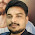Engineering Mechanics MCQ - Set 03 - ObjectiveBooks

# Practice Test: Question Set - 03

1. The Cartesian equation of trajectory is (where u = Velocity of projection, α = Angle of projection, and xy = Co-ordinates of any point on the trajectory after t seconds.)
(A) y = (gx²/2u² cos²α) + x. tanα
(B) y = (gx²/2u² cos²α) - x. tanα
(C) y = x. tanα - (gx²/2u² cos²α)
(D) y = x. tanα + (gx²/2u² cos²α)

2. The units of moment of inertia of an area are
(A) kg-m²
(B) m⁴
(C) kg/m²
(D) m³

3. The slope on the road surface generally provided on the curves is known as
(A) Angle of friction
(B) Angle of repose
(C) Angle of banking
(D) None of these

4. A force acting on a body may
(A) Change its motion
(B) Balance the other forces acting on it
(C) Retard its motion
(D) All of the above

5. The centre of gravity of an isosceles triangle with base (p) and sides (q) from its base is
(A) [√(4p² - q²)]/6
(B) (4p² - q²)/6
(C) (p² - q²)/4
(D) (p² + q²)/4

6. On a ladder resting on smooth ground and leaning against vertical wall, the force of friction will be
(A) Towards the wall at its upper end
(B) Away from the wall at its upper end
(C) Upwards at its upper end
(D) Downwards at its upper end

7. The velocity of a body on reaching the ground from a height h, is
(A) 2.√(gh)
(B) √(gh)
(C) √(2gh)
(D) 2g.√h

8. A framed structure, as shown in the below figure, is a

(A) Perfect frame
(B) Deficient frame
(C) Redundant frame
(D) None of the above

9. Lami's theorem states that
(A) Three forces acting at a point will be in equilibrium
(B) Three forces acting at a point can be represented by a triangle, each side being proportional to force
(C) If three forces acting upon a particle are represented in magnitude and direction by the sides of a triangle, taken in order, they will be in equilibrium
(D) If three forces acting at a point are in equilibrium, each force is proportional to the sine of the angle between the other two

10. The ratio of static friction to dynamic friction is always
(A) Equal to one
(B) Less than one
(C) Greater than one
(D) None of these

11. Which is the correct statement about law of polygon of forces?
(A) If any number of forces acting at a point can be represented by the sides of a polygon taken in order, then the forces are in equilibrium
(B) If any number of forces acting at a point can be represented in direction and magnitude by the sides of a polygon, then the forces are in equilibrium
(C) If a polygon representing forces acting at a point is closed then forces are in equilibrium
(D) If any number of forces acting at a point can be represented in direction and magnitude by the sides of a polygon taken in order, then the forces are in equilibrium

12. Moment of inertia of a triangular section of base (b) and height (h) about an axis through its base, is
(A) bh3/4
(B) bh3/8
(C) bh3/12
(D) bh3/36

13. Coefficient of friction is the
(A) Angle between normal reaction and the resultant of normal reaction and the limiting friction
(B) Ratio of limiting friction and normal reaction
(C) The friction force acting when the body is just about to move
(D) The friction force acting when the body is in motion

14. The forces, which meet at one point and their lines of action also lie on the same plane, are known as
(A) Coplanar concurrent forces
(B) Coplanar non-concurrent forces
(C) Non-coplanar concurrent forces
(D) Non-coplanar non-concurrent forces

15. A flywheel on a motor goes from rest to 1000 rpm in 6 sec. The number of revolutions made is nearly equal to
(A) 25
(B) 50
(C) 100
(D) 250

Show and hide multiple DIV using JavaScript View All Answers

Blogger Comment
1.